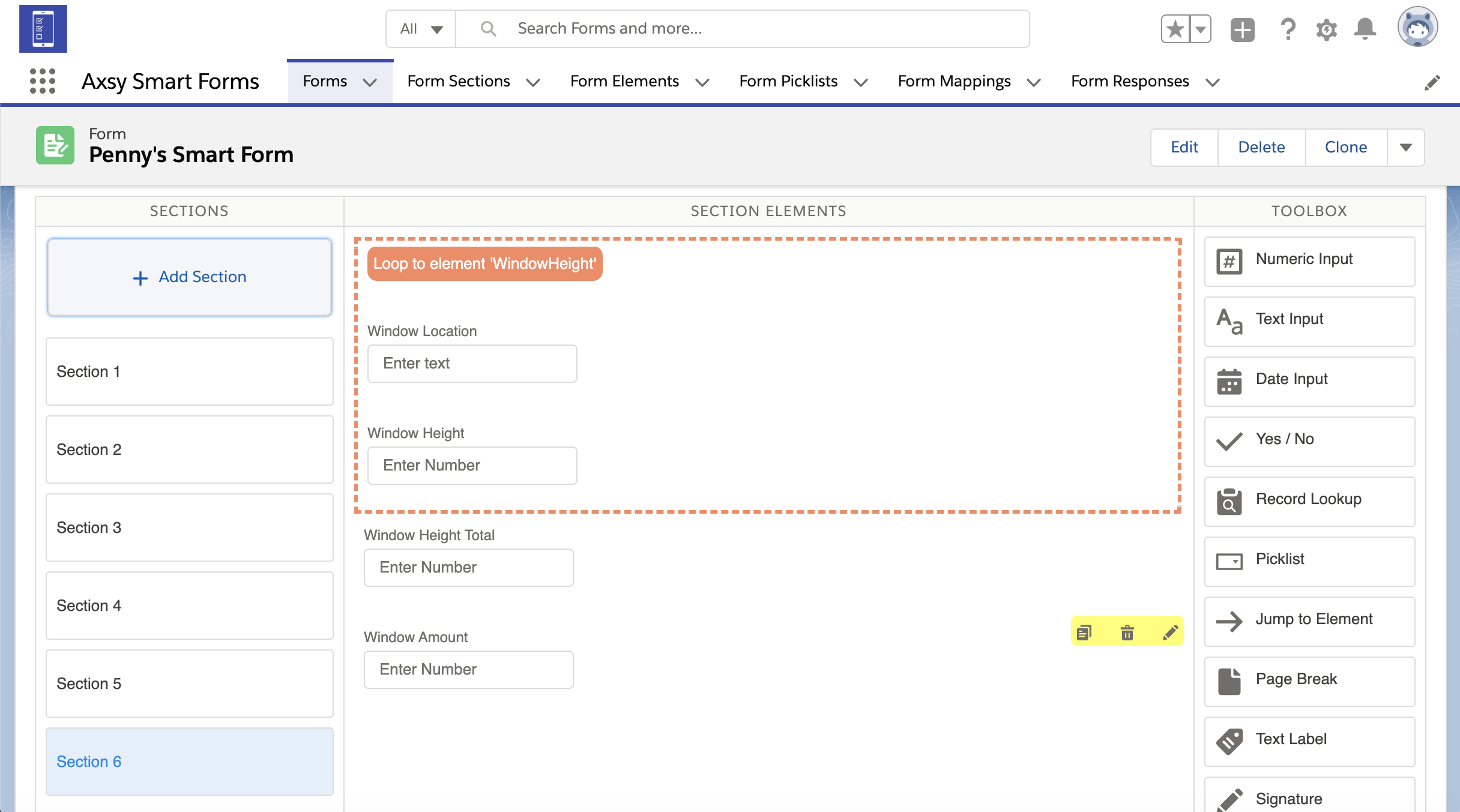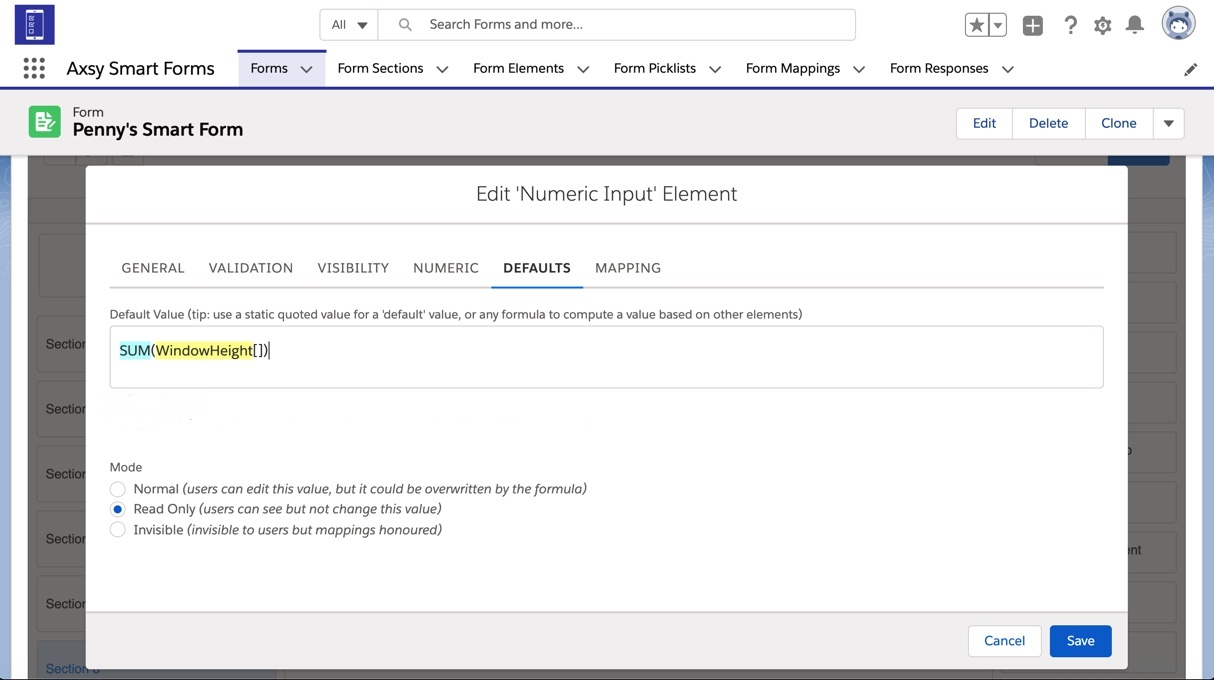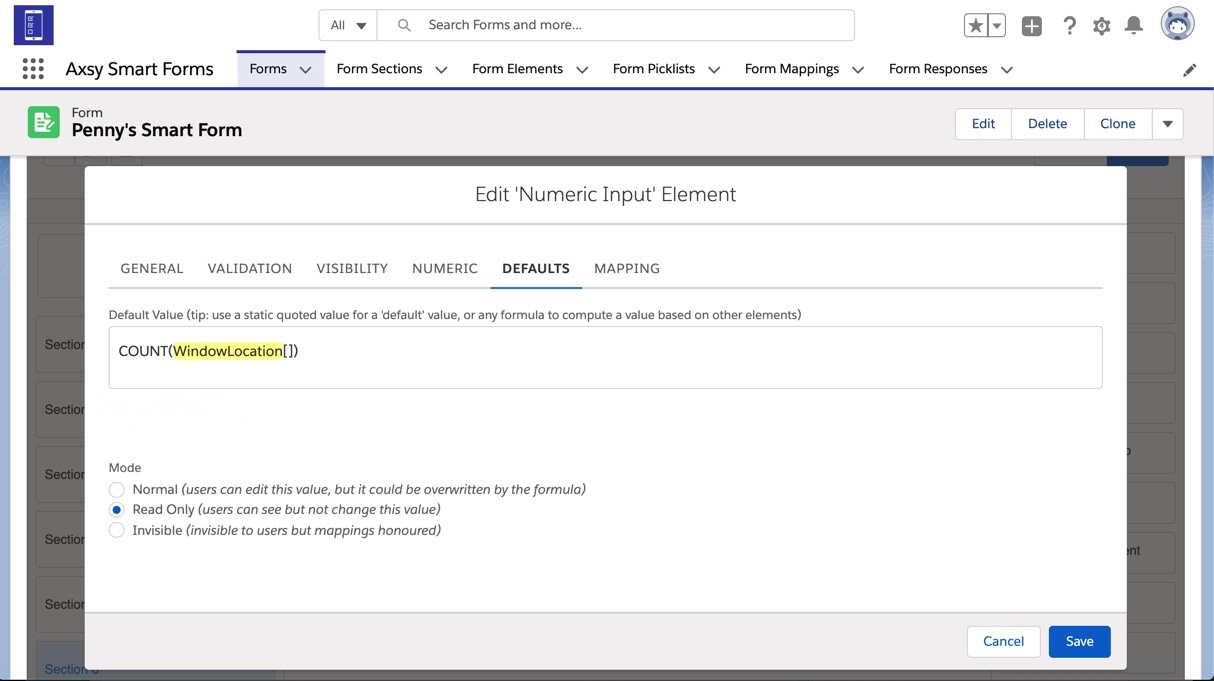Available in: Axsy Mobile for Salesforce, Axsy Field Service, Axsy Public Sector, Axsy Retail Execution

It is possible to calculate SUM and COUNT totals for the accumulation of a specific Element in a Loop. For more information on Loop Elements, please see this article: Configuring Loops

Elements that come after a Loop Element can make use of these roll-up calculations in their formulas by using the following syntax: SUM(ElementName[]) and COUNT(ElementName[]), where ElementName refers to an Element encapsulated by a Loop Element that needs to be summed or counted - Don't forget the square brackets []!

It should also be noted that these formulas only work with specific Element Types:

• SUM works only with Numeric Elements
• COUNT works with Text, Numeric, Picklist and Date Elements

# Example Roll-Up Formulas

Here is a Loop about windows. This example Form includes:

• Two Elements within the Loop that contain the window height and location: WindowHeight and WindowLocation.
• Two Elements outside of the Loop for calculating the total number of windows and total height of all of the windows: TotalWindowCount and TotalWindowHeight.To find the total SUM of the height of the windows, you can use a formula for the Default Value of the TotalWindowHeight Element and reference the WindowHeight Element field encapsulated by the Loop Element. Use the Formula: SUM(WindowHeight[]) to add up all the values entered in the WindowHeight Element field for all loop iterations and populate a total.You can find the total COUNT of the amount of windows data that has been recorded for by referencing any of the elements within the Loop. Use the formula: COUNT(WindowLocation[]) to count the number of times WindowLocation has been displayed in the Loop and populate how many windows there are in total.